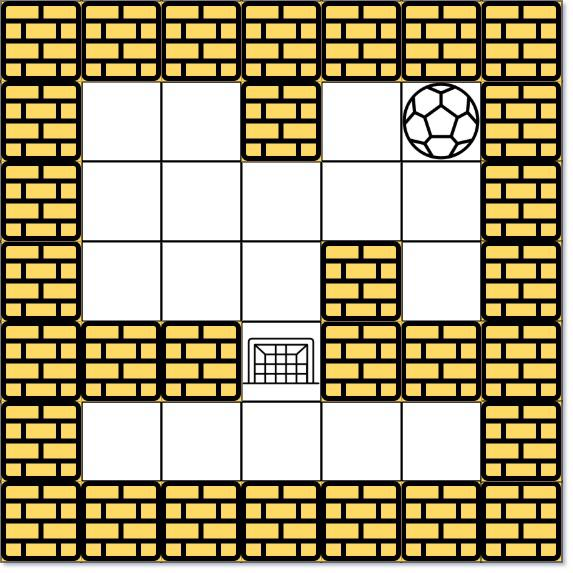By LongLuo

# 参考文献

By Long Luo

490. 迷宫 Medium
505. 迷宫 II Medium
499. 迷宫 III Hard

# 490. The MazeBy Long Luo

By Long Luo

# 252. 会议室

## 排序

By Long Luo

Here shows 4 Approaches to slove this problem: Two Pointers, Sorting, Priority Queue and Binary Search with Two Pointers.

# Two Pointers

## Analysis

• Time Complexity: $O(n + klogk)$.
• Space Complexity: $O(n)$.

By Long Luo

Here shows 3 Approaches to slove this problem: Brute Force, Stack, Slow Fast Pointers.

# Brute Froce

It’s easy to think of the way that we traverse the linked list first, from the head node to the end node, to get the length of the linked list $L$.

Then we traverse the linked list from the head node again. When the $L-n+1$ th node is traversed, it is the node we need to delete.

We can traverse $L-n+1$ nodes starting from the dummy node. When traversing to the $L-n+1$th node, its next node is the node we need to delete, so we only need to modify the pointer once to complete the deletion operation.

## Analysis

• Time Complexity: $O(n)$
• Space Complexity: $O(n)$

By Long Luo

# IntuitionIt’s like Leetcode 11. Container With Most Water , we can consider the black block as a wall, the blue block as water. Given an array, each element represents the height of the wall from left to right, find out how many units of water can be held.

The solution can be refered 2 Approaches: BF and Two Pointers with Image Explaination .

# 1. Brute Force

It’s easy to use the brute force approach. The time complexity is $O(max^n)$, so it will TLE.

## Analysis

• Time Complexity: $O(max^n)$
• Space Complexity: $O(1)$

By Long Luo

By Long Luo

By Long Luo

0%